# Match Cut-offs

## Scores for Core and Matrix Similarity

The matrix similarity (MSS) is a score that describes the quality of a match between a matrix and an arbitrary part of the input sequences. Analogously, the core similarity (CSS) denotes the quality of a match between the core sequence of a matrix, i.e. the five most conserved positions within a matrix, and a part of the input sequence. The matrix similarity score (and analogously the core similarity score) for a subsequence of length L is calculated according to the following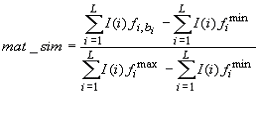where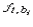is the frequency of nucleotide b at position i of the matrix with width L,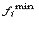the frequency of the rarest occurring nucleotide in position i, and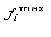the frequency of the most frequent occurring nucleotide in position i. The information vector I(i) describes the conservation of nucleotide B in position i of the matrix: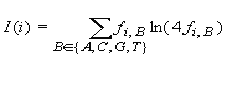, i = 1, 2, ..., L

A match/site has to contain the "core sequence" of a matrix, i.e. the core sequence has to match with a score higher than or equal to the core similarity cut-off. In addition, only the matches that score higher than or equal to the selected matrix similarity cut-off appear in the output.

## Cut-off to Minimize False Negative Matches (minFN)

The false negative rate was measured, as far as available, on known genomic binding sites for the transcription factors associated with the matrix. In case not sufficient (less than 10) genomic binding sites were available, SELEX sites or sets of generated oligonucleotides were used for estimating the cut-offs to minimize the false negative rate, using actual weight matrices to calculate the probability of a nucleotide occurring at a certain position of a binding site. For each matrix we applied the Match algorithm to these test sequence sets without using any matrix similarity cut-offs. Then we set the cut-off to a value that provides recognition of at least 90% of oligonucleotides. We decided to tolerate an error rate of ten percent (FN10). We call this set of cut-offs minFN cut-offs.

Applying the minFN cut-offs, the user will find most genomic binding sites, but in this case a high rate of false positives should be taken into account as well. The minFN cut-offs are useful for the detailed analysis of relatively short DNA fragments.

## Cut-off to Minimize False Positive Matches (minFP)

To estimate the number of false positives found by Match, we applied the Match algorithm to upstream sequences (-10,000 to -5,000 relative to the virtual TSS) from TRANSPRO. That score that gives 1% of hits in these sequences relative to the number of hits received when using the minFN score (calculated above) is defined as minFP.

When a minFP cut-off is applied for searching a DNA sequence, the algorithm will find a relatively low number of matches per nucleotide. In the output, the user will only find putative sites with a good similarity to the weight matrix; however, some known genomic binding sites could not be recognized. This kind of cut-off is useful, for example, for searching the most promising potential binding sites in extended genomic DNA sequences.

## Cut-off to Minimize the Sum of Both Error Rates (minSUM)

To minimize the sum of both error rates, false positives and false negatives, we compute the number of matches found in promoter sequences for each matrix using a cut-off allowing 10% of false negative matches (FN10 = minFN). This cut-off is defined as 100% of false positives. The sum of corresponding percentages for false positives and false negatives is then computed for every cut-off ranging from minFN to minFP. The cut-off that gives the minimum sum is then referred as the minSUM cut-off.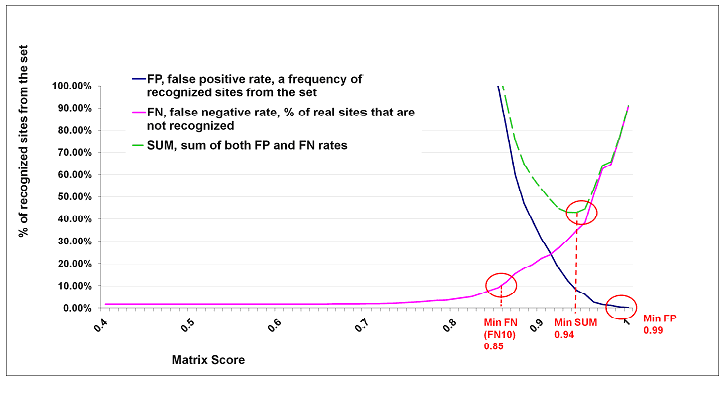## Cut-offs for Various False Negative Rates

In addition to the three cut-offs described above (minFN, minFP, minSUM) further cut-offs are provided in the Profile Generation tool of Match, for false negative rates of 10% (FN10), 30% (FN30), 50% (FN50), 70% (FN70), 90% (FN90). The false negative rate was measured as described above for minFN (= FN10). For each of these false negative rates, also the number of false positives (based on upstream sequences) is provided in the Profile Generation tool.

### FN10

This cut-off allows a false negative rate of 10%. Note: minFN and FN10 are identical.

### FN30

This cut-off allows a false negative rate of 30%.

### FN50

This cut-off allows a false negative rate of 50%.

### FN70

This cut-off allows a false negative rate of 70%.

### FN90

This cut-off allows a false negative rate of 90%.

### Other Cut-offs

You can also use other matrix or core similarity scores as cut-offs on the Match input page or when you generate a profile in the Profile Generation tool.

Please note: We use the term "profile" for a specific subset of weight matrices from the TRANSFAC® library with core similarity cut-off values and matrix similarity cut-off values for each matrix.

## Matrix Quality

Matrices producing more than 10 hits (false positives) per 1000 nucleotides (in sequences, 10,000 to 5,000 nucleotides upstream of the transcription start sites) at minSUM are defined as "low quality matrices". These matrices, about 5% of the current matrices, give about 50% of all false positive hits. By using the "Use only high-quality matrices" option in the Match input page these matrices can be excluded from a Match analysis. For user generated matrices, however, always all matrices which were selected during profile generation are used.

## Sites search optimization with F-Match algorithm

The F-Match algorithm compares the number of sites found in a query sequence set (experimental set or Yes set) against the background set (No set). It is assumed, if a certain transcription factor (TF), or factor family, plays a significant role in the regulation of the considered set of promoters, then the frequency of the corresponding sites found in these sequences should be significantly higher than expected by random chance. Often, the stringency of the interaction of this TF with their target sequences in the considered promoters is not known, leading to the uncertainty in setting thresholds on the site searches using the Match program.

F-Match evaluates the set of promoters, and for each matrix tries to find two thresholds: one, th-max, which provides the maximum ratio between the frequency of matches in the promoters in focus (query set) and background promoters (background set) (over-represented sites); and the second threshold, th-min, that minimizes the same ratio (underrepresented sites). As a result, for each weight matrix we obtain a set of predicted K sites and M sites in the both promoter sets with the corresponding matrix scores.

The F-Match algorithm makes an exhaustive search through the space of all scores observed in the sequence sets (all sites above the selected primary Match cut-off). Each observed score is taken as a threshold th and the program computes the number of sites k found in the main promoter set and number of sites m found in the background promoter set. Then, the expected number of sites in the main set to be observed in the case of even distribution of sites between two sets will be: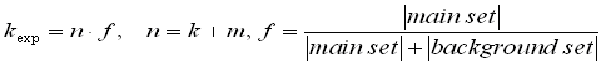and assuming a binomial distribution of the sites between two sets, we can calculate the p-value of finding the observed number of sites and higher, for over-represented matches, or lower, in the case of under-represented matches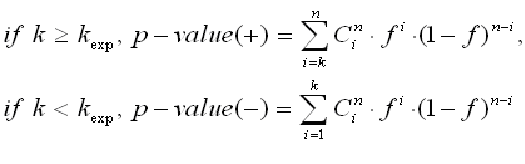giving the p-value of over- and under-representation of matches in the main promoter set.

For a given significance level p (e.g. p = 0.01), F-Match finds such thresholds th-max and th-min that maximizes and minimizes, respectively, the ratio k/kexp provided that the p-value < p. If the required significance level cannot be reached for a given matrix, this matrix will not be considered.

Thus, the matrices and number of matches/sites in the F-Match result are based on the optimized Match cut-offs (MSS and CSS) and the selected p-value threshold.